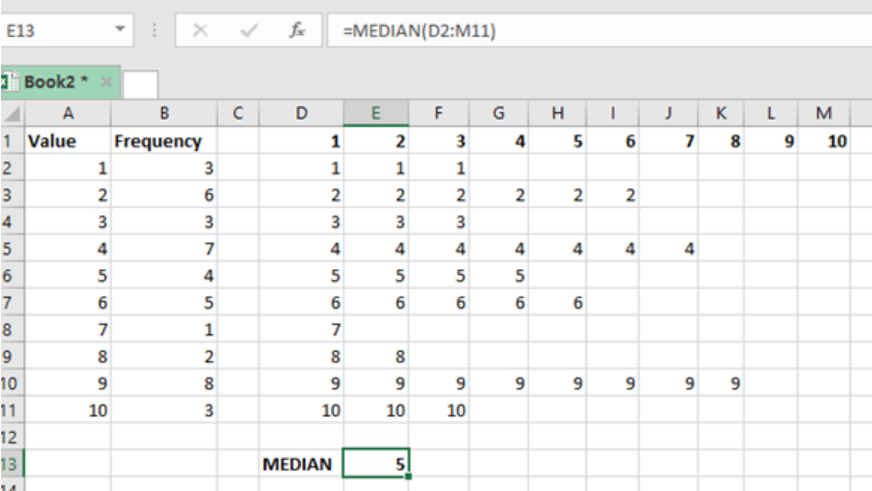Get instant live expert help with Excel or Google Sheets“My Excelchat expert helped me in less than 20 minutes, saving me what would have been 5 hours of work!”

#### Post your problem and you’ll get expert help in seconds.

Your message must be at least 40 characters
Our professional experts are available now. Your privacy is guaranteed.

# Learn How to Calculate the Median from a Frequency Table in Excel

Calculating the median from a frequency table is not as straightforward as many would want to think. Note that when working with a frequency table, the numbers might be repeating themselves a number of times and thus this might make it a little bit hard for us to get the median.

It is good to remember that the median is the middle number in a given list of numbers. If the total number is odd, then the median will be the middle one. But if the list is an even number, then we shall have to take n/2 + (n+1)/2 to get the median.

## But how do you get the median when you have a frequency table?

This post provides an easy way on how one can get the median from a frequency table.Figure 1. Final result

## Procedure

• For us to get the median of values in a frequency table, we first need to tabulate our table with the values and the number of times the values occur. This is as shown in figure 1 above where we have the values in column A and their frequencies in column B.
• After that, we need to get the maximum number in the array so that we can have columns equal the total number of values in the list. This is as shown from cell D1:M10. Notice that the max value is 10.
• We then have the following formula in cell D2;
`=IF(D\$1>\$B2,"",\$A2)`
• We then copy this formula across the entire range, from cell D2:M11. This will bring each individual number and not as a frequency.
• After that, we use the normal excel MEDIAN function to get the median of the array of values as shown below;
`=MEDIAN(D2:M11)`

## Instant Connection to an Expert through our Excelchat Service

Most of the time, the problem you will need to solve will be more complex than a simple application of a formula or function. If you want to save hours of research and frustration, try our live Excelchat service! Our Excel Experts are available 24/7 to answer any Excel question you may have. We guarantee a connection within 30 seconds and a customized solution within 20 minutes.

Solution examplesNeed to calculate out two equally spaced points between a range of dates. Basically like the MEDIAN function between two cells that have dates, but I need to produce two equally spaced points between that range. For example. If I have the following date range (A1) 2/2/2018 (B1) 7/2/2018, then that's roughly 150 days total. I want 4 equally spaced points (Start, Start-Mid, Mid-End, End). So I'd say that's 50 day chunks spaced euqally. Which would produce 4 total dates 2/2/2018 - 3/24/2018 - 5/13/2018 - 7/2/2018.
Solved by K. J. in 20 minsi have this formula currently which works but not if I want it for every day - any ideas ?? (NETWORKDAYS.INTL(L1362,M1362,11,BH!A:A)-1)*("17:30"-"8:30")+IF(NETWORKDAYS.INTL(M1362,M1362,11,BH!A:A),MEDIAN(MOD(M1362,1),"8:30","17:30"),"17:30")-MEDIAN(NETWORKDAYS.INTL(L1362,L1362,11,BH!A:A)*MOD(L1362,1),"8:30","17:30")
Solved by A. D. in 28 mins